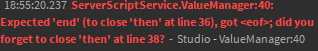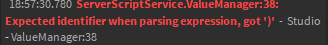# Timer Output Error Confusing

So… I tried making the timer, but in the ValueManager it gives me an error.

If i dont put close the “then” at line 38 and the “end” at line 40, it gives me this error:But if i close the “then” at line 38 and the “end” at line 40, it gives me this error:The last portion of the script should be

``````VCE.OnServerEvent:Connect(function(Player, TriggeredValue, Died)
print(TriggeredValue, Died)
if Died == "SetTime" then
local Timer = Player:FindFirstChild("PlayerGui"):FindFirstChild("Timer")
if Timer then
Timer.TextLabel.Text = TriggeredValue
end
end
end)
``````